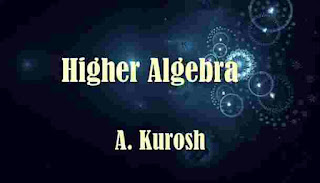# Higher Algebra by A. Kurosh (1984) PDF ebook

## Higher Algebra by A. Kurosh (1984)Higher Algebra

The education of the mathematics major begins with the study of three basic disciplines: mathematical analysis, analytic: geometry, and higher algebra.

These disciplines have a number of points of contact, some of which overlap; together they constitute the foundation upon which rests the whole edifice of modern mathematical science. Higher algebra—the subject of this text—is a far-reaching and natural generalization of the basic school course of elementary algebra.

Central to elementary algebra is without doubt the problem of solving equations. The study of equations begins with the very simple case of one equation. of the first degree in one unknown. From there on, the development proceeds in two directions: to systems of two and three equations of the first degree in two and, respectively, three unknowns, and to a single quadratic equation in one unknown and also to a few special types of higher-degree equations which readily reduce to quadratic equations (quartic equations, for example).

Both trends are further developed in the course of higher algebra, thus determining its two large areas of study. One—the foundations of linear algebra—starts with the study of arbitrary systems of equations of the first degree (linear equations).

When the number of equations equals the number of unknowns, solutions of such systems are obtained by means of the theory of determinants. Howe- ver, the theory proves insufficient when studying systems of linear equations in which the number of equations is not equal to the number of unknowns. This is a novel feature from the standpoint of elementary algebra, but it is very important in practical applications.

This stimulated the development of the theory of matrices, which are systems of numbers arranged in a square or rectangular arrays made up of rows and columns. Matrix theory proved to be very deep and has found application far beyond the limits of the theory of systems of linear equations. On the other hand, investigations into systems of linear equations gave rise to multidimensional (so-called vector or linear) spaces.

To the nonmathematician, multidimensional space (four-dimensional, to begin with) is a nebulous and often confusing concept. Actually, however, the notion is a strictly mathematical one, mainly algebraic, and serves as an important tool in a variety of mathematical investigations and also in physics. The second half of the course of higher algebra, called the algebra of polynomials, is devoted to the study of a single equation in one unknown but of arbitrary degree. Since there is a formula for solving quadratic equations, it was natural to seek similar formulas for higher-degree equations. \

That is precisely how this division of algebra developed historically. Formulas for solving equations of third and fourth-degree were found in the sixteenth century. The search was then on for formulas capable of expressing the roots of equations of a fifth and higher degree in terms -of the coefficients of the equations by means of radicals, even radicals within radicals.

It was futile, though it continued up to the beginning of the nineteenth century when it was proved that no such formulas exist and that for all degrees beyond the fourth there even exist specific examples of equations with integral coefficients roots that cannot be written down by means of radicals, One should not be saddened by this absence al formulas for solving equations of higher degrees, for even in the case of third and fourth-degree equations, where such formulas exist, computations are extremely involved and, in a practical sense, almost useless.

On the other hand, the coefficients of equations one encounters in physics and engineering are usually quantities obtained in measurements. These are approximations and therefore the roots need only be known approximately, to within a specified accuracy. This led to the elaboration of a variety of methods of approximate solution of equations; only the most elementary methods are given in the course of higher algebra. However, in the algebra of polynomials the main thing is not the problem of finding the roots of equations, but the problem of their existence. For example, we even know of quadratic equations with real coefficients that do not have real-valued roots.

By extending the range of numbers to include the collection of complex numbers, we find that quadratic equations do have roots and that this holds true for equations of the third and fourth degree as well, as follows from the existence of formulas for their solution. But perhaps there are equations of the fifth and higher degree without a single root even in the class of complex numbers. Will, it not be necessary, when seeking the roots of such equations, to pass from complex numbers to a still bigger class of numbers?

The answer to this question is contained in an important theorem which asserts that any equation with numerical coefficients, whether real or complex, has complex-valued (real-valued, as a special case) roots; and, generally speaking, the number of roots is equal to the degree of the equation.